# Calderón-Zygmund operator

An operatordefined on a space of sufficiently smooth functionswith compact support in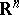by the formula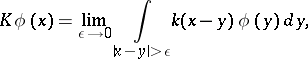where the kernelis a homogeneous function of degree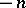with zero mean value over the unit sphere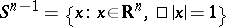. The kernelhas the form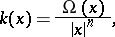where, the characteristic function of, satisfies the conditions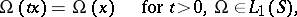(*)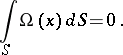The Calderón–Zygmund operator is usually written in the formhere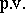denotes the principal value of the integral. In the one-dimensional case the Calderón–Zygmund operator becomes the Hilbert operator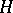:The Calderón–Zygmund operator can be extended by continuity to the space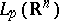of functionsinthat are summable of degree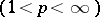. This extension mapscontinuously into itself. Ifsatisfies the condition (*) and also Dini's condition: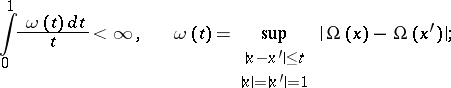and if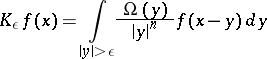for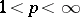and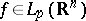, then

a) there exists a constant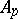(independent ofor) such that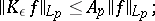b) the limitexists in the sense of convergence in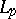and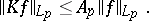The Calderón–Zygmund operator was analyzed by A.P. Calderón and A. Zygmund .